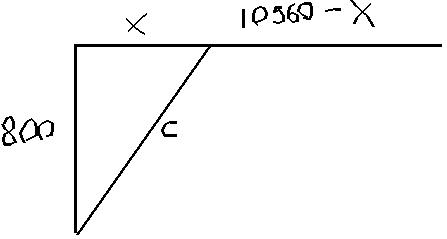# Dayton Power and Light, Inc., has a power plant on the Miami Riverwhere the river is 800 ft wide. To lay a new cable from the plantto a location in thpermaneceerc 2021-02-14 Answered
Dayton Power and Light, Inc., has a power plant on the Miami Riverwhere the river is 800 ft wide. To lay a new cable from the plantto a location in the city 2 mi downstream on the opposite sidecosts $180 per foot across the river and$100 per foot along theland.
(a) Suppose that the cable goes from the plant to a point Q on theopposite side that is x ft from the point P directly opposite theplant. Write a function C(x) that gives the cost of laying thecable in terms of the distance x.
(b) Generate a table of values to determin if the least expensivelocation for point Q is less than 2000 ft or greater than 2000 ftfrom point P.
You can still ask an expert for help

• Questions are typically answered in as fast as 30 minutes

Solve your problem for the price of one coffee

• Math expert for every subject
• Pay only if we can solve ittabuordy
First lets put the two distances the cable will cover in terms of x:The length on the ground is 2 miles minus x. to find c weneed to use the pythagorean theorem:
${800}^{2}+{x}^{2}={c}^{2}$
$c=\sqrt{{800}^{2}-{x}^{2}}$
now we have all the information we need to make a costfunction:
$C\left(x\right)=180\sqrt{{800}^{2}-{x}^{2}}+100\left(10560-x\right)$
b) use point plotting to answer b